# GPU 原生推理¶

```wget https://paddle-inference-dist.bj.bcebos.com/Paddle-Inference-Demo/resnet50.tgz
```

## 1. C++ 示例¶

C++ 示例代码在链接，你可以将此仓库全部克隆下来留着使用。下面从先介绍 Paddle Inference C++ API 的使用流程，然后介绍在 Linux/Ubuntu 系统下和 Windows 系统下编译和执行此示例代码。

(1) 包含头文件

```#include "paddle/include/paddle_inference_api.h"
```

(2) 设置 Config

Config 默认用 CPU 推理，若要用 GPU，需手动开启，设置分配的初始显存 和 运行的 GPU 卡号。同时可以设置开启 IR 优化、开启内存优化、开启 TensorRT 加速等。Paddle Inference 中启用 TensorRT 的相关说明和示例可以参考文档

```paddle_infer::Config config;
config.SetModel(FLAGS_model_file, FLAGS_params_file); // Load combined model
config.EnableUseGpu(500, 0);
config.SwitchIrOptim(true);
config.EnableMemoryOptim();
config.EnableTensorRtEngine(1 << 30, FLAGS_batch_size, 10, PrecisionType::kFloat32, false, false);
```

(3) 创建 Predictor

```std::shared_ptr<paddle_infer::Predictor> predictor = paddle_infer::CreatePredictor(config);
```

(4) 设置输入

```auto input_names = predictor->GetInputNames();
auto input_t = predictor->GetInputHandle(input_names);
std::vector<int> input_shape = {1, 3, 224, 224};
std::vector<float> input_data(1 * 3 * 224 * 224, 1);
input_t->Reshape(input_shape);
input_t->CopyFromCpu(input_data.data());
```

(5) 执行Predictor

```predictor->Run();
```

(6) 获取输出

```auto output_names = predictor->GetOutputNames();
auto output_t = predictor->GetOutputHandle(output_names);
std::vector<int> output_shape = output_t->shape();
int out_num = std::accumulate(output_shape.begin(), output_shape.end(), 1,
std::multiplies<int>());
std::vector<float> out_data;
out_data.resize(out_num);
output_t->CopyToCpu(out_data.data());
```

### Linux/Ubuntu 部署示例¶

```WITH_MKL=ON
WITH_GPU=ON
USE_TENSORRT=OFF

CUDNN_LIB=/usr/lib/x86_64-linux-gnu/
CUDA_LIB=/usr/local/cuda/lib64
TENSORRT_ROOT=/usr/local/TensorRT-7.1.3.4
```

```bash compile.sh
bash run.sh
```

### Windows 部署示例¶

• 选择源代码路径，及编译产物路径，如图所示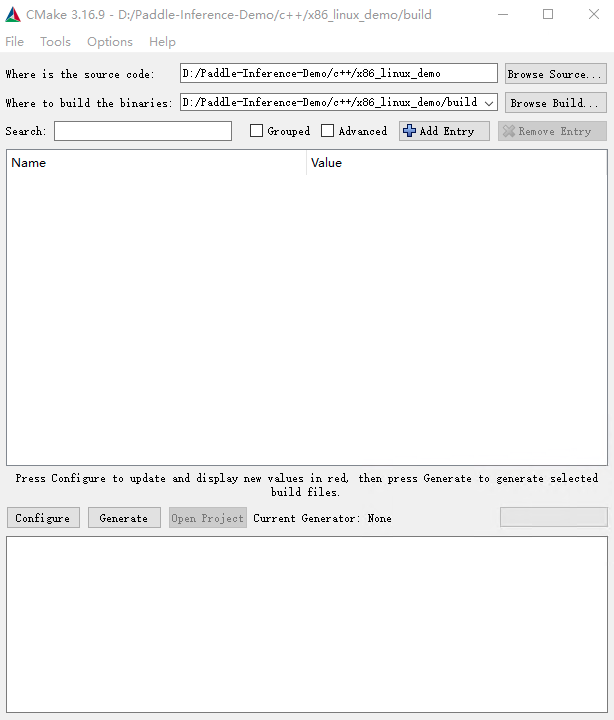win_x86_cpu_cmake_1

• 点击 Configure，选择 Visual Studio 且选择 x64 版本如图所示，点击 Finish，由于我们没有加入必要的 CMake Options，会导致 configure 失败，请继续下一步。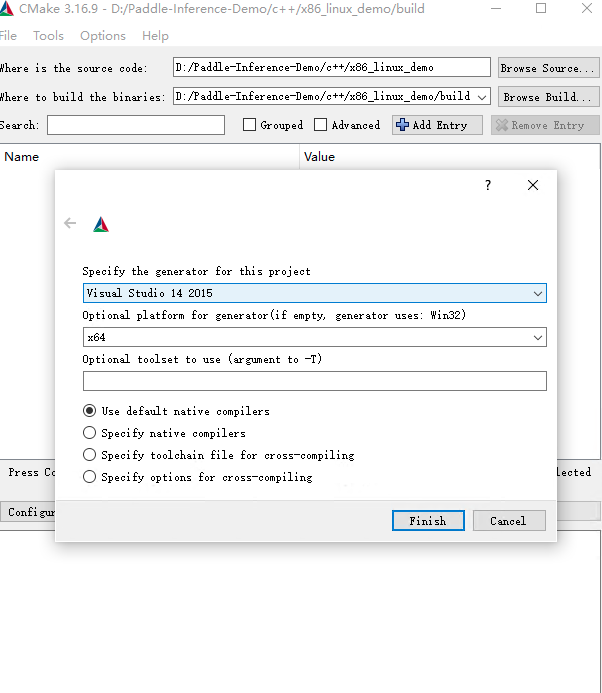win_x86_cpu_cmake_2

• 设置 CMake Options，点击 Add Entry，新增 PADDLE_LIB、CMAKE_BUILD_TYPE、DEMO_NAME等选项。具体配置项如下图所示，其中 PADDLE_LIB 为您下载的推理库路径，DEMO_NAME 设置为`.cc`文件的文件名。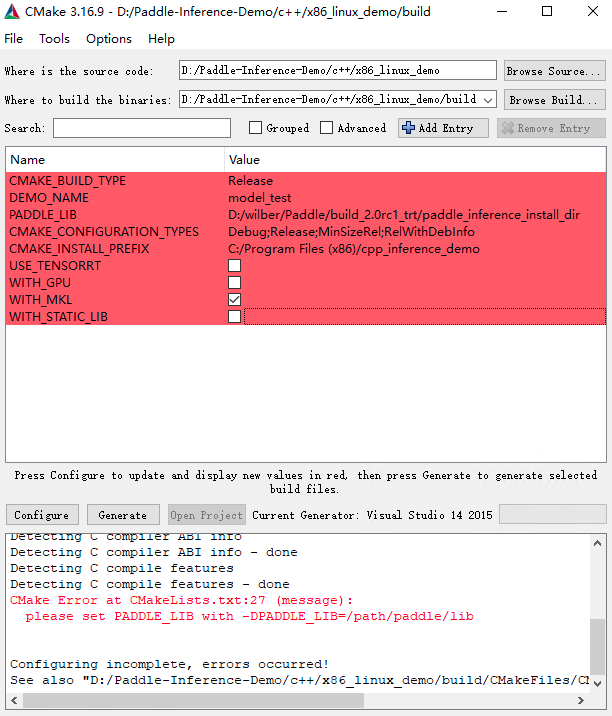win_x86_cpu_cmake_3

• 点击 Configure，log 信息显示 Configure done 代表配置成功，接下来点击 Generate 生成vs工程，log 信息显示 Generate done，代表生成成功，最后点击 Open Project 打开 Visual Studio。

• 设置为 Release/x64，编译，编译产物在 build/Release 目录下。win_x86_cpu_vs_1

• 首先设置 model_test 工程为启动首选项。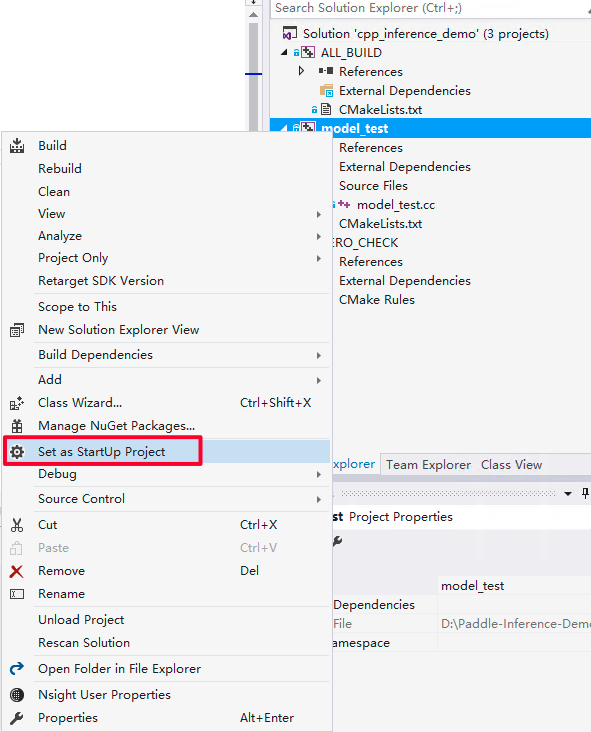win_x86_cpu_vs_2

• 配置输入 flags，即设置您之前下载的模型路径。点击 Debug 选项卡的`model_test Properities..`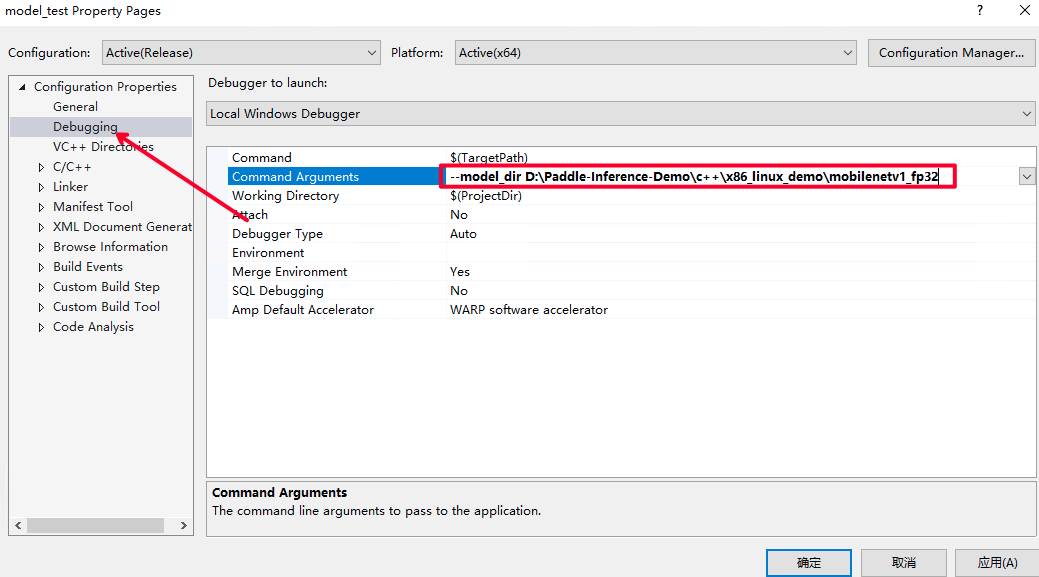win_x86_cpu_vs_3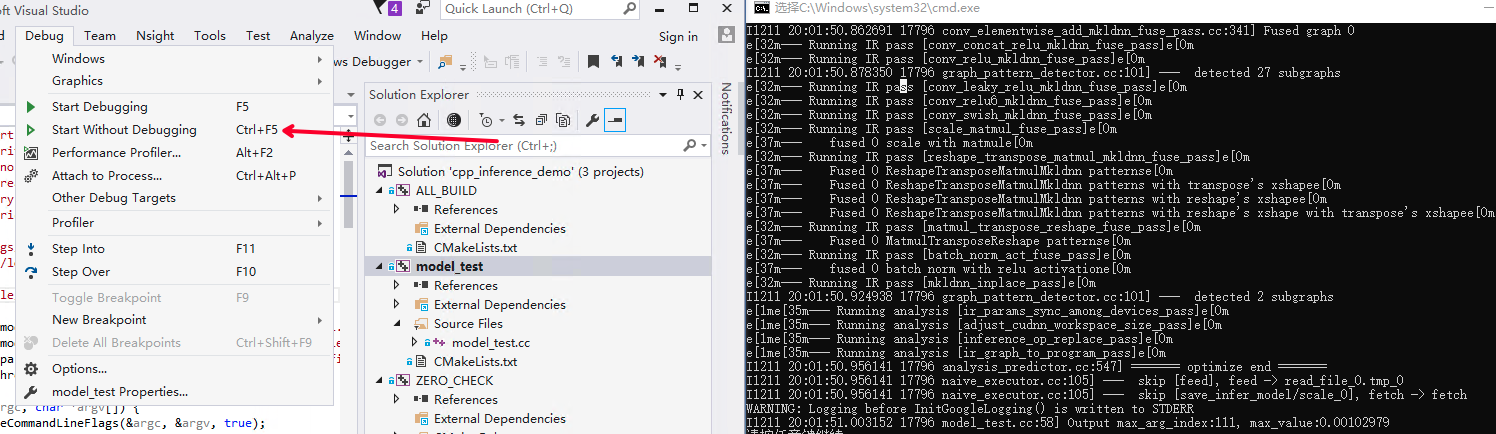win_x86_cpu_vs_4

## 2. Python 示例¶

Python 示例代码在链接，下面从先介绍 Paddle Inference Python API 的使用流程，然后介绍在 Linux/Ubuntu 系统下和 Windows 系统下编译和执行此示例代码。

（1） Python 导入

```from paddle.inference import Config
```

（2）设置 Config

Config 默认用 CPU 推理，若要用 GPU 推理，需手动开启，设置分配的初始显存 和 运行的 GPU 卡号。同时可以设置开启 IR 优化、开启内存优化、开启 TensorRT 加速等。Paddle Inference 中启用 TensorRT 相关说明和示例可以参考文档

```# args 是解析的输入参数
# Init config
config = Config(args.model_file, args.params_file)
config.enable_use_gpu(500, 0)
config.switch_ir_optim()
config.enable_memory_optim()
config.enable_tensorrt_engine(workspace_size=1 << 30, precision_mode=PrecisionType.Float32,max_batch_size=1, min_subgraph_size=5, use_static=True, use_calib_mode=False)
```

（3）创建Predictor

```# Create predictor
predictor = create_predictor(config)
```

（4） 设置输入

```img = cv2.imread(args.img_path)
img = preprocess(img)
input_names = predictor.get_input_names()
input_tensor = predictor.get_input_handle(input_names)
input_tensor.reshape(img.shape)
input_tensor.copy_from_cpu(img.copy())
```

（5） 执行 Predictor

```predictor.run()
```

（6） 获取输出

```output_names = predictor.get_output_names()
output_tensor = predictor.get_output_handle(output_names)
output_data = output_tensor.copy_to_cpu()
```